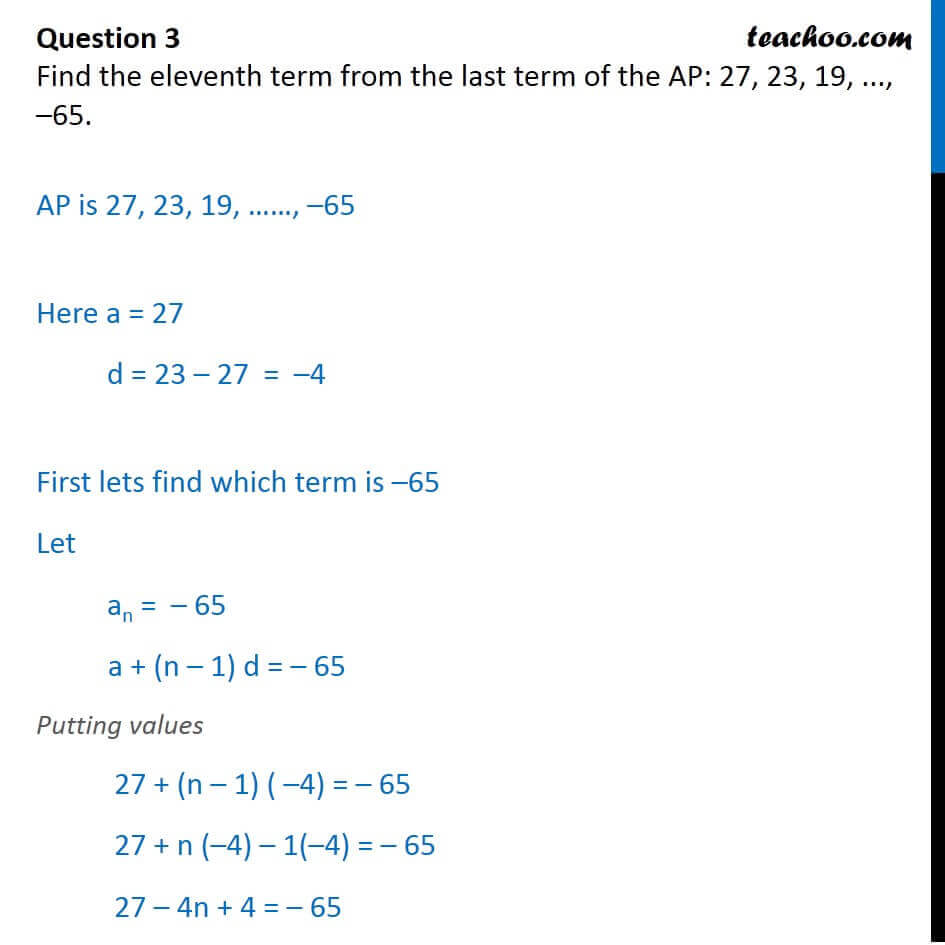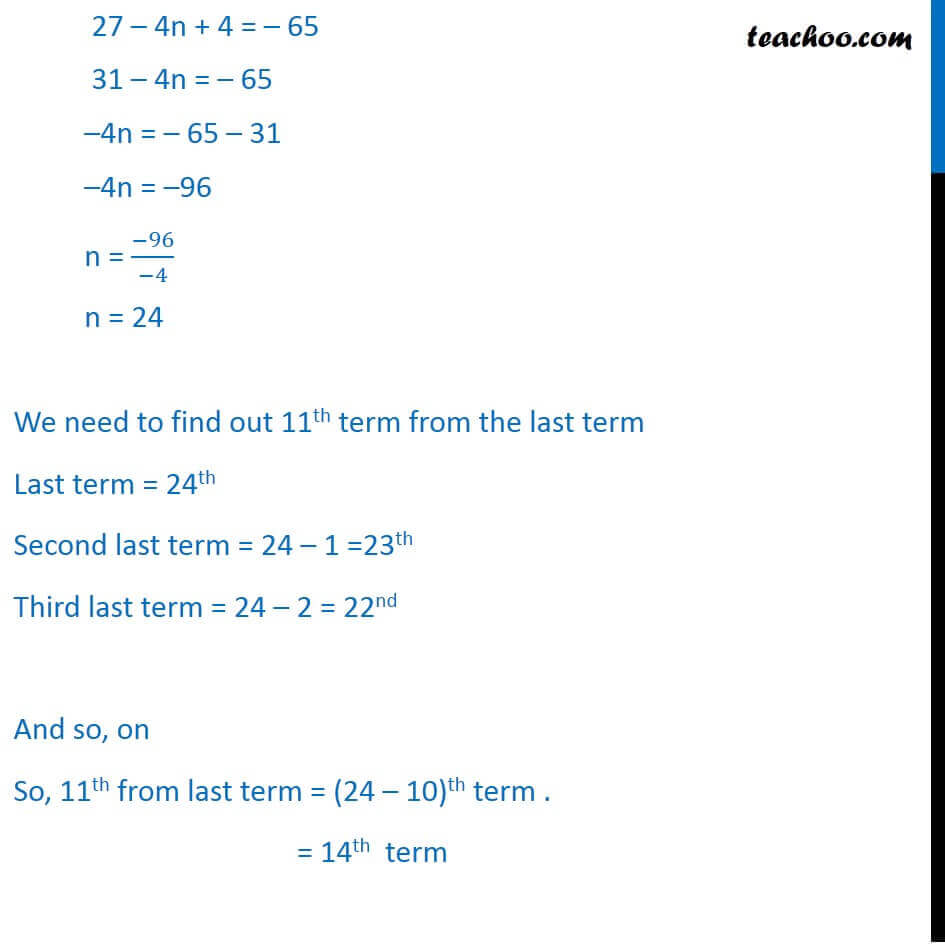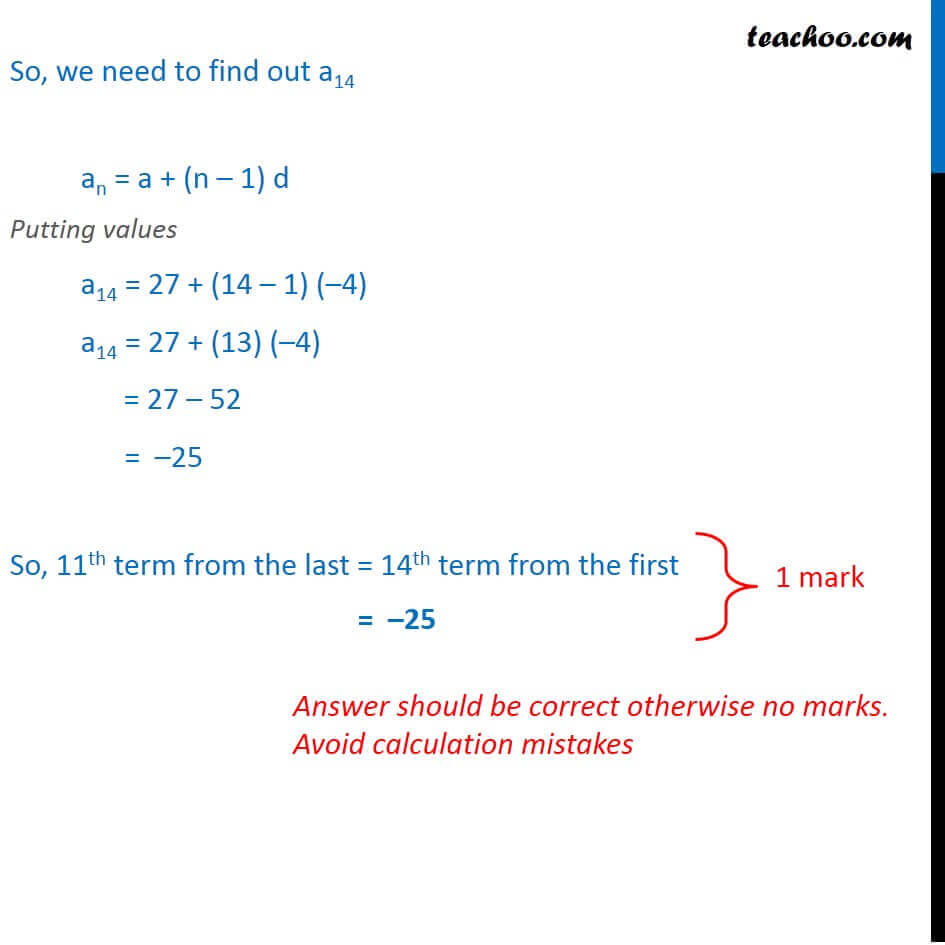CBSE Class 10 Sample Paper for 2018 Boards

Class 10
Solutions of Sample Papers for Class 10 Boards

### Find the eleventh term from the last term of the AP: 27, 23, 19, ..., –65.

This is a question of CBSE Sample Paper - Class 10 - 2017/18.Learn in your speed, with individual attention - Teachoo Maths 1-on-1 Class

### Transcript

Question 3 Find the eleventh term from the last term of the AP: 27, 23, 19, ..., 65.AP is 27, 23, 19, , 65 Here a = 27 d = 23 27 = 4 First lets find which term is 65 Let an = 65 a + (n 1) d = 65 Putting values 27 + (n 1) ( 4) = 65 27 + n ( 4) 1( 4) = 65 27 4n + 4 = 65 27 4n + 4 = 65 31 4n = 65 4n = 65 31 4n = 96 n = ( 96)/( 4) n = 24 We need to find out 11th term from the last term Last term = 24th Second last term = 24 1 =23th Third last term = 24 2 = 22nd And so, on So, 11th from last term = (24 10)th term . = 14th term So, we need to find out a14 an = a + (n 1) d Putting values a14 = 27 + (14 1) ( 4) a14 = 27 + (13) ( 4) = 27 52 = 25 So, 11th term from the last = 14th term from the first = 25